# C Program to Calculate Average and Percentage Marks

In this article, you will learn and get code about how to create a program that finds averages and percentage marks. The last program given here is the complete version of finding the average and percentage marks of a student. In that program, the number of subjects and maximum marks are also decided by the user.

## Find the average marks in C

The question is, "Write a program in C that asks the user to enter marks obtained in five subjects and prints the average mark as output." So the answer to this question is given below:

```#include<stdio.h>
#include<conio.h>
int main()
{
int i;
float mark, sum=0, avg;
printf("Enter Marks obtained in 5 Subjects: ");
for(i=0; i<5; i++)
{
scanf("%f", &mark);
sum = sum+mark;
}
avg = sum/5;
printf("\nAverage Mark = %0.2f", avg);
getch();
return 0;
}```

This program was compiled and executed in the Code::Blocks IDE. This is the first snapshot of the sample run: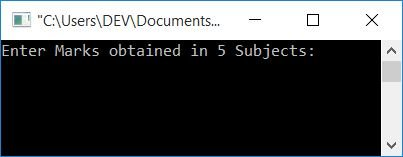Now supply the marks obtained in 5 subjects as input, say 76, 87, 98, 70, and 82, and press the ENTER key to see the average mark of the student as shown in the second snapshot of the sample run given below: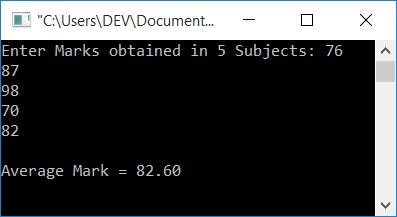#### Steps used in previous program

Here are some of the main steps used in the above program:

• Declare a variable, say i of int type to work with it in a loop.
• Declare another three variables: mark, sum, and avg of type float.
• Here these three variables are declared as "float," because to hold any value that contains decimal
• Initialize 0 to the variable sum in the declaration part.
• Now here we have applied a for loop that runs five times.
• The program scans the mark value and adds it to the sum variable at each run.
• So after exiting from the loop, we have a sum variable that holds the sum of all the 5 marks entered by the user at run-time.
• Simply divide the sum by 5 to get the average of the five numbers.
• Print the value of avg as output.

## Find the percentage mark in C

The question is: write a program in C that asks the user to enter marks obtained in five subjects (out of 100) and finds the percentage of marks obtained by the student. The answer to this question is given below:

To calculate the percentage marks of a student in C programming, you have to ask the user to enter marks obtained in some number of subjects, say 5. Put the sum of 5 marks in a variable called sum, and the sum/5 in a variable called avg. Simply print "avg" as the result on the output.

```#include<stdio.h>
#include<conio.h>
int main()
{
int i;
float mark, sum=0, perc;
printf("Enter Marks obtained in 5 Subjects (Out of 100): ");
for(i=0; i<5; i++)
{
scanf("%f", &mark);
sum = sum+mark;
}
perc = (sum/500)*100;
printf("\nPercentage Mark = %0.2f%c", perc, 37);
getch();
return 0;
}```

This program will produce the output as shown in the snapshot given below: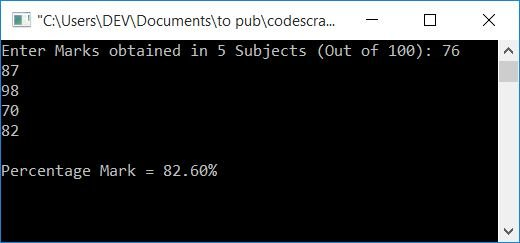Most of the code written in this program is almost the same as the previous program except that we used the %c format specifier with 37 as its value. Because the %c format specifier is used to print characters and the ASCII value of the % character is 37, So to print the % sign on the output screen after the percentage value, we have used its ASCII value as shown in the above output.

## Calculate the Average and Percentage Mark in C

The question is: write a program in C that asks the user to enter marks obtained and finds the average and percentage mark value. Here the subject count and the maximum marks must be entered by the user. The answer to this question is given below.

This is the complete version of the calculating average and percentage marks program in C. Here, the number of subjects and maximum mark are decided by the user. Let's have a look at it:

```#include<stdio.h>
#include<conio.h>
int main()
{
int i, n, max;
float mark, sum=0, avg, perc;
printf("How many Subject runs in your Institute ? ");
scanf("%d", &n);
printf("\nEnter Maximum Mark of Subject: ");
scanf("%d", &max);
printf("\nEnter Marks obtained in %d Subjects (Out of %d): ", n, max);
for(i=0; i<n; i++)
{
scanf("%f", &mark);
sum = sum+mark;
}
avg = sum/n;
perc = (sum/(n*max))*100;
printf("\nAverage Mark = %0.2f", avg);
printf("\nPercentage Mark = %0.2f%c", perc, 37);
getch();
return 0;
}```

The snapshot given below shows the initial output of this program: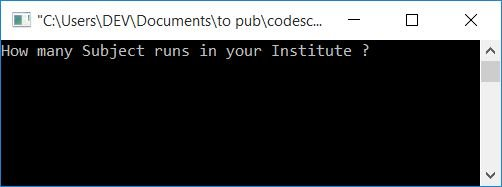And the snapshot given below shows the final output of this program after supplying the total number of subject runs in the institute, maximum marks, and marks obtained in all subjects.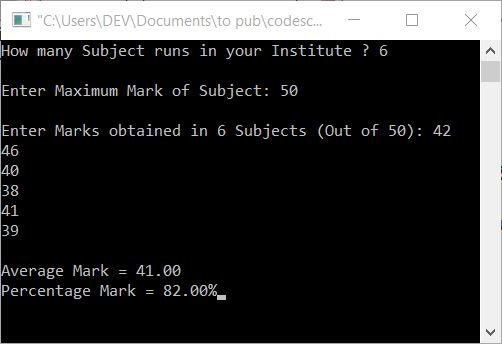Let's look at the above program (and its output) through the lens of the following example:

• The user enters 6 as the first question, which is, "How many subjects run in your institute?" So n holds its value of 6.
• The user enters 50 as the second question. So the variable max has a value of 50.
• Now the user enters the marks obtained in each subject one by one. And the summation operation is performed on each scan like this:
• On the first scan, the user enters 42, so sum+mark or 0+42 (0 is the initial value of sum) is initialized to sum.
• The value of sum is now 42.
• On the second scan, the user enters 46, so 42+46 or 88 is initialized to sum.
• The value of sum is now 88.
• The user enters 40 on the third scan, so 88+40 is set to sum.
• The value of sum is now 128.
• On the fourth scan, the user enters 38, so 128+38 or 166 is initialized to sum.
• sum now has 166 as its value.
• On the fifth scan, the user enters 41, so 166+41 or 207 is initialized to sum.
• The value of sum is now 207.
• The user enters 39 on the sixth scan, so 207+39 or 246 is initialized to sum.
• The value of sum is now 246.
• As of now, sum contains 246; n contains 6; and max contains 50.
• So, sum/n or 246/6 or 41 gets initialized to the avg variable.
• And, (sum/(n*max))*100 or (246/(6*50))*100 or (246/300)*100 or 82 gets initialized to the perc variable.
• Now simply print the value of the avg and perc variables as output for average and percentage marks.
• %0.2f is used to print values up to 2 decimal places only. Because 37 is the ASCII value of the % sign, %c with a value of 37 is used to print the % sign on the output.

#### The same program in different languages

C Quiz

« Previous Program Next Program »